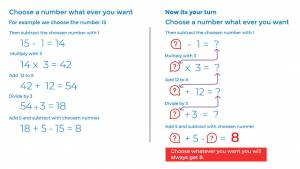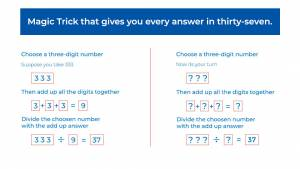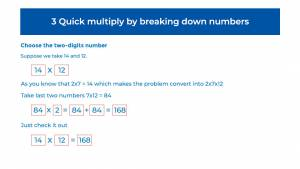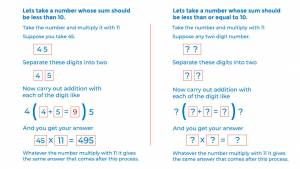# Genius Mathematics Tricks That Truly Help Every Child

Maths is a subject that usually feels tough to kid. Many kids find it difficult to grip the core of mathematics principles which can make them smart to solve sums.  Mathematics tricks for those kids may help to catch the grip on those principles, understands techniques and make their skills boost up. And for few people, they grow out with their special skills of maths after that tricks.

You surely have to use these tricks to make your kid’s interest in maths.

## Top 5 Mathematics Tricks For Kids

### 1. Choose Whatever You Want, You Will Always Get Eight

This method is a unique and interesting method that attracts kids to experience their own work on it. This method will work on any number. Let’s try this:

• Start choosing with the number… 15
• Now you have to subtract one from this number… 15-1=14
• Multiply the answer with three… 14×3=42
• Add twelve to it… 42+12=54
• When you divided by three it looks… 54/3=18
• Now simply just add five in it and subtract from the actual number… 18+5-15=8

Isn’t it amazing…### 2. A Magic Trick That Gives You Every Answer In Thirty-Seven

A cool magic trick that makes your kid go to a large number. It always gives you an answer in 37. That the magic thing. Now try this with other kids too…

• You must choose a three-digit number for this trick.
• Start this with using 333
• Then add up all the digits together… 3+3+3=9
• Divide the number with 333. So it looks like… 333/9=37
• Yes, you got this. Simple and fascinating.\3. Remember tables give you grip on all term of maths:

Tables the term that is the root of max ¼ math. Teach your kids the table in fun ways. Go sit with them and let them watch the table song and make them pick it up high. Learning tables with the fun way is always easy ad helpful for kids. They catch it like they are playing and then they boost their skills on it. So these mathematics tricks will help your child remember tables and make them grip on all terms of maths.### 3. Quick Multiply By Breaking Down Numbers

Multiplication is nothing. it is just a simple and easy way of mathematics. it is a combination of multiple additions.

Let’s try:

• Starting with the number of two digits.
• Take 14×12.
• As you know that 2×7 is 14. which makes the problem convert into 2x7x12.
• Try it with the last two numbers 7×12 =84
• 84×2=84+84=168
• Just check it out 14×12 is also equal to 168.### 4. Find An Answer By Just Multiplying It To Eleven

Most kids do just end up memorizing tables till 10. But this mathematics tricks will let your child know tables further and quick.

• Let’s try this procedure with multiplying with 45 with 11
• Separate these digits into two and then put space into it… 4[]5
• Now carry out addition with each of the digit like… 4[4+5=9]5.